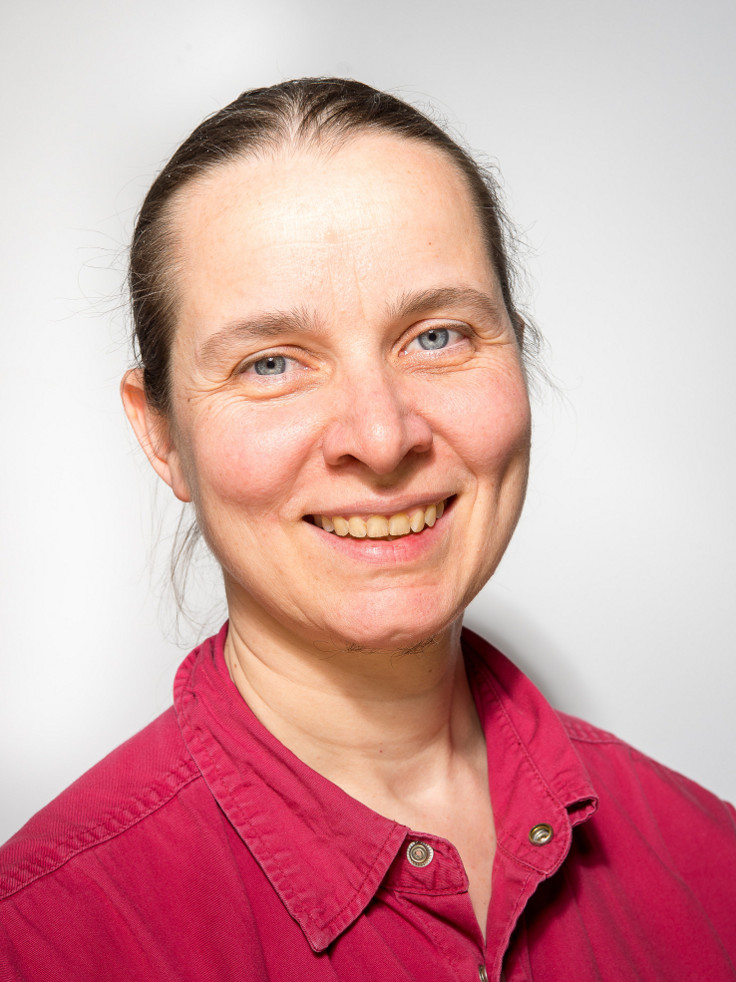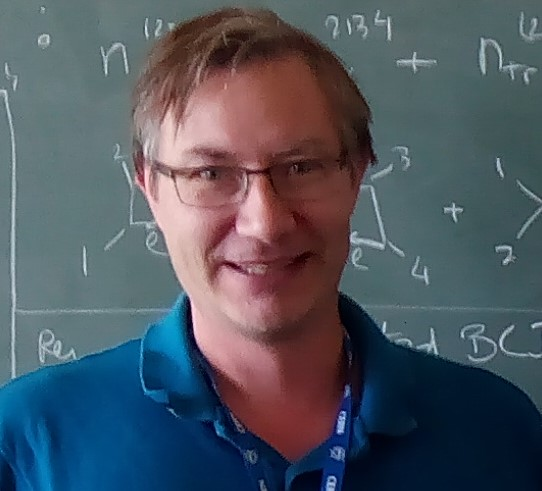# Tutorial Speakers

## Titles and Abstracts

Janko Böhm
Universität Kaiserslautern, Germanyand
Anne Frühbis-Krüger
Carl von Ossietzky Universität, Germany### Massively parallel computations in algebraic geometry

Abstract:

Introducing parallelism and exploring its use is still a fundamental goal for the computer algebra community. In high performance numerical simulation, transparent environments for distributed computing which follow the principle of separating coordination and computation have been a success story for many years. Recently, this approach has turned out to be also successful in computer algebra, for example, for handling complicated parallel structures arising in geometry, which are based on local to global principles for schemes and sheaves. Exploiting parallelism of this kind so far was a significant challenge due to the unpredictability of Buchberger's algorithm.

In this tutorial, we introduce the audience to the use of the Petri net based workflow management system GPI-Space in conjunction with the computer algebra system Singular as frontend and the computational backend. We start out by illustrating how to use the Singular/GPI-Space framework for realizing basic programming constructs. We will then discuss various applications with an in depth discussion of one of them, the verification of smoothness of an algebraic scheme based on a criterion of Hironaka. In this application parallelism arises through a tree of charts built by the algorithm.

Marc Moreno Maza### Design and implementation of multi-threaded algorithms in polynomial algebra

Abstract:

Since the early 2000s, the omnipresence of parallel architectures and memory hierarchies has led to a new quest for parallel algorithms and software capable of exploiting various levels and schemes of parallelism. Computer Algebra has a long history with parallelism, where the research community has been exploring the parallelization of high-level algorithms (e.g. Gröbner bases and cylindrical algebraic decomposition) since the 1980s, before shifting its attention to low-level algorithms (e.g. polynomial and matrix arithmetic) as multicore processors became widely available.

Often computer algebraists extract parallelism from a serial divide-and-conquer algorithm (e.g. dense matrix multiplication or Cooley-Tukey FFT) but make little use of other parallelism patterns (e.g. pipelines and stencils). Also, optimizing memory usage and memory access patterns (e.g. minimizing cache misses) and optimizing scheduling costs are often considered only at the implementation stage and not at the stage of algorithm design, which can result in missing opportunities (say w.r.t. portability or scalability).

In this tutorial, we will discuss techniques that help in the design of multi-threaded algorithms as well as tools for implementing those algorithms. Our targeted application area is polynomial algebra, considering both low-level and high-level algorithms.

We will start by illustrating the importance of analyzing (theoretically and practically) memory access patterns. We will present techniques for reducing memory footprint.

A second part will be dedicated to parallel patterns, emphasizing the fact that these algorithmic strategies should be understood together with the support that the targeted concurrency platform provides for those patterns. Examples of parallel patterns suitable for polynomial algebra are: divide-and-conquer, workpile and pipeline.

A third part will tackle the question of identifying parallel patterns. A popular instance of that question is whether a given for-loop nest can be modified (e.g. through a change of coordinates) to obtain a nest where some of the for-loops can be executed in parallel.

The last part of this tutorial will deal with the software implementation of the support for various parallel patterns often provided by concurrency platforms. Challenges and solutions will be illustrated with the BPAS library.

Pierre Vanhove
CEA, France### Differential equations for Feynman integrals

Abstract:

Feynman integrals enter the evaluation of many physical observables in particle physics, gravitational physics, statistical physics and solid-state physics. They are multidimensional integrals which cannot be evaluated with elementary methods. It is an important question to understand what kind of special functions they are of their physical parameters.

In this tutorial we will present algebraic geometrical approaches for determining the Feynman integral D-modules. We will first introduce the parametric representations of a generic Feynman integral, and explain that Feynman integrals are holonomic functions, and that their ideal of annihilators generates a holonomic D-module. Then we will present a derivation of the holonomic D-module using the creative telescoping algorithm. We will then analyse the relation between this D-module and the generalized Gel'fand-Kapranov-Zelevinsky D-module obtained from a toric geometrical approach to the Feynman integrals. This tutorial will be illustrated by several examples.